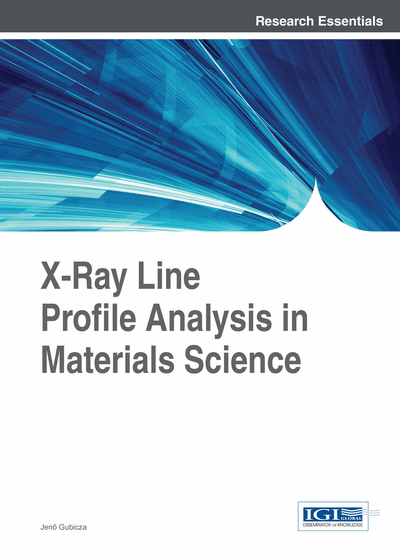Line Profiles Caused by Planar Faults

DOI: 10.4018/978-1-4666-5852-3.ch004
Available
\$37.50
No Current Special Offers

Abstract

The planar faults in crystalline materials yield characteristic broadening of X-ray line profiles. The diffraction peak shape caused by intrinsic and extrinsic stacking faults and twin boundaries formed on close packed {111} planes in face centered cubic (fcc) crystals are calculated. The Bragg reflections consist of subreflections that can be categorized by specific selection rules for the hkl indices. The breadth and the position of the subreflections relative to the exact Bragg angle depend on their indices. For instance, if the sum of indices of a subreflection is a multiple of three, neither the position nor the breadth of this peak is influenced by planar faults. Other subreflections are broadened and shifted simultaneously due to intrinsic and extrinsic stacking faults. For both fcc and hexagonal close packed (hcp) crystals each subreflection caused by twin boundaries is a sum of symmetric and antisymmetric Lorentzian functions. The latter profile component is caused by the interference between the radiations scattered from the parent and twinned lamellae in the crystal. The antisymmetric Lorentzian function yields a shift of the subprofile center. For fcc materials this displacement of peak position is marginal since twin boundaries are formed on close packed {111} planes; however in hcp crystals, where twinning usually occurs on pyramidal planes, this effect should be taken into account in the line profile evaluation. The effect of anti-phase boundaries on line profiles of superstructure reflections for Cu3Au is also discussed in this chapter.
Chapter Preview
Top

Introduction

Planar faults are two dimensional lattice defects in crystalline materials. As examples, Figure 1 shows three types of planar faults in face centered cubic (fcc) crystal structures, namely intrinsic and extrinsic stacking faults, as well as coherent twin boundary. These defects are formed on {111} planes in fcc crystals. When {111} planes are packed on each other in the three dimensional lattice, there are three different positions in the stacking sequence which are usually denoted by letters A, B and C. The perfect order of layers in an fcc lattice is ABCABC etc., which is altered when planar faults are formed. Figure 1 shows the stacking sequence for perfect order and the three types of planar faults. In the case of an intrinsic stacking fault a part of the lattice is shifted by vector

Figure 1.

The stacking sequence of {111} planes for perfect order of layers and the three types of planar faults in fcc crystals: intrinsic stacking fault, extrinsic stacking fault and coherent twin boundary. The three different positions of {111} planes are denoted by letters A, B and C. The dashed lines indicate the shift by vectorwhen the faults are formed.

along a {111} plane, resulting in change of plane positions from A to B, from B to C and from C to A in the displaced part of the lattice. The resulted stacking sequence is displayed in Figure 1b which is the same as if a {111} plane is removed from the lattice (layer B in Figure 1b). In the case of an extrinsic stacking fault a part of the lattice is shifted by vector along a {111} plane, and then with the same vector again along the neighboring {111} plane, as shown in Figure 1c. The stacking sequence for this fault is the same as if a close-packed plane is inserted into the lattice along a {111} plane (layer C in Figure 1c). This planar fault has a finite extension with a thickness of one layer perpendicular to {111} planes, as illustrated in Figure 1c (Kovács & Zsoldos, 1973). If the lattice is shifted by vector sequentially along each neighboring {111} plane in a part of the crystal, the boundary layer between the displaced and undisplaced volumes is a coherent twin boundary (see Figure 1d). The twin boundary is a mirror plane of the two halves of the crystal. In hexagonal close packed (hcp) crystals the shear of the crystal during twinning occurs mainly on {101}, {112}, {102} and {111} planes in,,anddirections, respectively. The first two and the last two twins are referred to as compressive and tensile twins, respectively. As an example, the twin boundary on plane {102} in a hcp crystal is illustrated in Figure 2. It is noted that in hcp crystals which have also an fcc allotropic phase (e.g. Co) planar faults are formed on {111} planes.

Complete Chapter List

Search this Book:
Reset